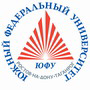Russian©  M. E. Abramyan (Southern Federal University, Shenzhen MSU-BIT University), 1998–2023

 Main Tasks Examples PT for MPI-2

 Overview Input-output operations Task groups Begin Integer Boolean If Case For While Series Proc Func Minmax Array Matrix String File Text Param Recur Dynamic Dynamic (obj) Tree Tree (obj)

# Integers

All input and output data are integer (i. e. whole numbers) in this group. All numbers with fixed amount of digits (for example, two-digit number, three-digit number and so on) are assumed to be positive.

Integer1°. A distance L is given in centimeters. Find the amount of full meters of this distance (1 m = 100 cm). Use the operator of integer division.

Integer2°. A weight M is given in kilograms. Find the amount of full tons of this weight (1 ton = 1000 kg). Use the operator of integer division.

Integer3°. A file size is given in bytes. Find the amount of full Kbytes of this size (1 K = 1024 bytes). Use the operator of integer division.

Integer4°. Two positive integers A and B are given (A > B). Segment of length A contains the greatest possible amount of inside segments of length B (without overlaps). Find the amount of segments B placed on the segment A. Use the operator of integer division.

Integer5°. Two positive integers A and B are given (A > B). Segment of length A contains the greatest possible amount of inside segments of length B (without overlaps). Find the length of unused part of the segment A. Use the operator of taking the remainder after integer division.

Integer6°. A two-digit integer is given. Output its left digit (a tens digit) and then its right digit (a ones digit). Use the operator of integer division for obtaining the tens digit and the operator of taking remainder for obtaining the ones digit.

Integer7°. A two-digit integer is given. Find the sum and the product of its digits.

Integer8°. A two-digit integer is given. Output an integer obtained from the given one by exchange of its digits.

Integer9°. A three-digit integer is given. Using one operator of integer division find first digit of the given integer (a hundreds digit).

Integer10°. A three-digit integer is given. Output its last digit (a ones digit) and then its middle digit (a tens digit).

Integer11°. A three-digit integer is given. Find the sum and the product of its digits.

Integer12°. A three-digit integer is given. Output an integer obtained from the given one by reading it from right to left.

Integer13°. A three-digit integer is given. Output an integer obtained from the given one by moving its left digit to the right side.

Integer14°. A three-digit integer is given. Output an integer obtained from the given one by moving its right digit to the left side.

Integer15°. A three-digit integer is given. Output an integer obtained from the given one by exchange a tens digit and a hundreds digit (for example, 123 will be changed to 213).

Integer16°. A three-digit integer is given. Output an integer obtained from the given one by exchange a ones digit and a tens digit (for example, 123 will be changed to 132).

Integer17°. An integer greater than 999 is given. Using one operator of integer division and one operator of taking the remainder find a hundreds digit of the given integer.

Integer18°. An integer greater than 999 is given. Using one operator of integer division and one operator of taking the remainder find a thousands digit of the given integer.

Integer19°. From the beginning of the day N seconds have passed (N is integer). Find an amount of full minutes passed from the beginning of the day.

Integer20°. From the beginning of the day N seconds have passed (N is integer). Find an amount of full hours passed from the beginning of the day.

Integer21°. From the beginning of the day N seconds have passed (N is integer). Find an amount of seconds passed from the beginning of the last minute.

Integer22°. From the beginning of the day N seconds have passed (N is integer). Find an amount of seconds passed from the beginning of the last hour.

Integer23°. From the beginning of the day N seconds have passed (N is integer). Find an amount of full minutes passed from the beginning of the last hour.

Integer24°. Days of week are numbered as: 0 — Sunday, 1 — Monday, 2 — Tuesday, …, 6 — Saturday. An integer K in the range 1 to 365 is given. Find the number of day of week for K-th day of year provided that in this year January 1 was Monday.

Integer25°. Days of week are numbered as: 0 — Sunday, 1 — Monday, 2 — Tuesday, …, 6 — Saturday. An integer K in the range 1 to 365 is given. Find the number of day of week for K-th day of year provided that in this year January 1 was Thursday.

Integer26°. Days of week are numbered as: 1 — Monday, 2 — Tuesday, …, 6 — Saturday, 7 — Sunday. An integer K in the range 1 to 365 is given. Find the number of day of week for K-th day of year provided that in this year January 1 was Tuesday.

Integer27°. Days of week are numbered as: 1 — Monday, 2 — Tuesday, …, 6 — Saturday, 7 — Sunday. An integer K in the range 1 to 365 is given. Find the number of day of week for K-th day of year provided that in this year January 1 was Saturday.

Integer28°. Days of week are numbered as: 1 — Monday, 2 — Tuesday, …, 6 — Saturday, 7 — Sunday. An integer K in the range 1 to 365 and an integer N in the range 1 to 7 are given. Find the number of day of week for K-th day of year provided that in this year January 1 was N-th day of week.

Integer29°. Three positive integers A, BC are given. A rectangle of the size A × B contains the greatest possible amount of inside squares with the side length C (without overlaps). Find the amount of squares placed on the rectangle and the area of unused part of the rectangle.

Integer30°. Given a year (as a positive integer), find the respective number of the century. Note that, for example, 20th century began with the year 1901.Designed byM. E. Abramyan and V. N. Braguilevsky Last revised:01.01.2023